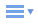# JavaScript Array 陣列

JavaScript Array 陣列是用來存取大量數據的好工具，Array 物件是 JavaScript 的內建物件，不需要額外安裝即可隨時調用，JavaScript Array 物件內可以存放其它物件或數值，用以管理大量數據時相當好用，可以運用建構子函數（constructor function)）來建立一個新的 Array 物件，同時或於建構完成後將 Array 值存入待用，其用法類似 PHP Array

<script type="text/javascript">
var ArrTest = new Array();　// 宣告一個新的陣列為 ArrTest
ArrTest = "A";　// 第一個陣列元素為 A
ArrTest = "B";　// 第二個陣列元素為 B
ArrTest = "C";　// 第三個陣列元素為 C
</script>

var ArrTest= ["A", "B", "C"];

var ArrTest= ["A", "B", "C"];
ArrTest='BBB';

<script type="text/javascript">
var ArrTest = new Array();
ArrTest = "A";
ArrTest = "B";
ArrTest = "C";

for(i=0;i<3;i++){
document.write(ArrTest[i]);
}
</script>

<script type="text/javascript">
var ArrTest = new Array();
ArrTest = "A";
ArrTest = "B";
ArrTest = "C";

var i=0;
while(i<3){
document.write(ArrTest[i]);
i++;
}
</script>